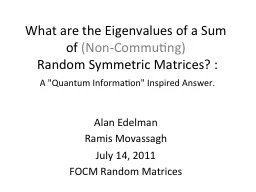# What are the Eigenvalues of a Sum ofEmbed code:

## What are the Eigenvalues of a Sum of

Download Presentation - The PPT/PDF document "What are the Eigenvalues of a Sum of" is the property of its rightful owner. Permission is granted to download and print the materials on this web site for personal, non-commercial use only, and to display it on your personal computer provided you do not modify the materials and that you retain all copyright notices contained in the materials. By downloading content from our website, you accept the terms of this agreement.

### Presentations text content in What are the Eigenvalues of a Sum of

Slide1

What are the Eigenvalues of a Sum of (Non-Commuting) Random Symmetric Matrices? : A "Quantum Information" Inspired Answer.

Alan Edelman

Ramis

Movassagh

July 14, 2011

FOCM

Random Matrices

Slide2

Example Resultp=1  classical probabilityp=0 isotropic convolution (finite free probability)

We call this “isotropic

entanglement”

Slide3

Simple Question

The

eigenvalues of

where the diagonals are random, and randomly ordered. Too easy?

Slide4

Another Question

where Q is orthogonal with Haar measure. (Infinite limit = Free probability)

The

eigenvalues of

T

Slide5

Quantum Information Question

where Q is somewhat complicated. (This is the general sum of two symmetric matrices)

The

eigenvalues of

T

Slide6

Preview to the Quantum Information Problem

mxm

nxn

mxm nxn

Summands commute,

eigenvalues

If A and B are random

eigenvalues

are

classical sum of random variables

Slide7

Closer to the true problem

d

2

xd2 dxd

dxd d2xd2

Nothing commutes,

eigenvalues

non-trivial

Slide8

Actual Problem Hardness =(QMA complete)

d

i-1xdi-1 d2xd2 dN-i-1xdN-i-1

The Random matrix could be

Wishart

,

Gaussian Ensemble, etc (Ind Haar Eigenvectors)The big matrix is dNxdN

Interesting Quantum Many Body System Phenomena tied to this overlap!

Slide9

Intuition on the eigenvectors

Classical Quantum Isostropic

Kronecker

Product of

Haar

Measures for A and B

Slide10

Moments?

Slide11

Matching Three Moments Theorem

Slide12

A first try:Ramis “Quantum Agony”

Slide13

The Departure Theorem

A “Pattern Match”

Hardest to Analyze

Slide14

The Istropically Entangled Approximation

But this one is hard

The kurtosis

Slide15

The convolutions

Assume A,B diagonal. Symmetrized ordering.A+B:A+Q’BQ:A+Qq’BQq

(“hats” indicate joint density is being used)

Slide16

The Slider Theorem

p only depends on the eigenvectors! Not the

eigenvalues

Slide17

Wishart

Slide18

Bernoulli ±1

Slide19

Slide20

Summary

Slide21

Slide22